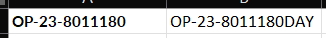cancel
Showing results for
Did you mean:

Fabric is Generally Available. Browse Fabric Presentations. Work towards your Fabric certification with the Cloud Skills Challenge.Helper I

## Excel formula to power query M code

Hi all, Im trying to convert the following formula in excel to M code :

=CONCATENATE(H2,
MID("ABCDEFGHIJKLMNOPQRSTUVWXYZ012345",(
IFERROR(IF(FIND(MID(H2,1,1),"ABCDEFGHIJKLMNOPQRSTUVWXYZ")>0,1,0),0)
+IFERROR(IF(FIND(MID(H2,2,1),"ABCDEFGHIJKLMNOPQRSTUVWXYZ")>0,2,0),0)

+IFERROR(IF(FIND(MID(H2,3,1),"ABCDEFGHIJKLMNOPQRSTUVWXYZ")>0,4,0),0)
+IFERROR(IF(FIND(MID(H2,4,1),"ABCDEFGHIJKLMNOPQRSTUVWXYZ")>0,8,0),0)
+IFERROR(IF(FIND(MID(H2,5,1),"ABCDEFGHIJKLMNOPQRSTUVWXYZ")>0,16,0),0)
+1),1),
MID("ABCDEFGHIJKLMNOPQRSTUVWXYZ012345",(
IFERROR(IF(FIND(MID(H2,6,1),"ABCDEFGHIJKLMNOPQRSTUVWXYZ")>0,1,0),0)
+IFERROR(IF(FIND(MID(H2,7,1),"ABCDEFGHIJKLMNOPQRSTUVWXYZ")>0,2,0),0)
+IFERROR(IF(FIND(MID(H2,8,1),"ABCDEFGHIJKLMNOPQRSTUVWXYZ")>0,4,0),0)
+IFERROR(IF(FIND(MID(H2,9,1),"ABCDEFGHIJKLMNOPQRSTUVWXYZ")>0,8,0),0)
+IFERROR(IF(FIND(MID(H2,10,1),"ABCDEFGHIJKLMNOPQRSTUVWXYZ")>0,16,0),0)
+1),1),
MID("ABCDEFGHIJKLMNOPQRSTUVWXYZ012345",(
IFERROR(IF(FIND(MID(H2,11,1),"ABCDEFGHIJKLMNOPQRSTUVWXYZ")>0,1,0),0)
+IFERROR(IF(FIND(MID(H2,12,1),"ABCDEFGHIJKLMNOPQRSTUVWXYZ")>0,2,0),0)

+IFERROR(IF(FIND(MID(H2,13,1),"ABCDEFGHIJKLMNOPQRSTUVWXYZ")>0,4,0),0)
+IFERROR(IF(FIND(MID(H2,14,1),"ABCDEFGHIJKLMNOPQRSTUVWXYZ")>0,8,0),0)
+IFERROR(IF(FIND(MID(H2,15,1),"ABCDEFGHIJKLMNOPQRSTUVWXYZ")>0,16,0),0)
+1),1))

Assuming, H2 is a 15 digit string of mix of letters and numbers.

Please can you help me out with this.

12 REPLIES 12Solution Sage

It looks like you are looking for the letters in your initial string. I put your formula into Excel. Can you exlpain how you get DAY suffix from that starting string (that only has two letters in it)?Pat

Microsoft EmployeeHelper I

Hi @ppm1 Apologies, I made a mistake in  my question. The original 15 digit string is a mix of letters and numbers.Solution Sage

Can you give an example of an input string with the expected output? The one shown isn't clear.

Pat

Microsoft EmployeeHelper I

@ppm1 hi, here is an example of the 15 digit string - 0062o00001CG0ObHelper I

hi @ppm1 do you have any solution or anywhere I can start ?Solution Sage

This is fairly complex but it does match the Excel result (when all the letters are upper case). Add a custom column and put the code below in the pop-up box, replacing TextColumn with the name of your text input column.

``````let
input = Text.Upper([TextColumn]),
letters = {"A".."Z"},
concatvalues = {null, "A".."Z", "0".."5"},
splitinput = List.Split(Text.ToList(input), 5),
lettercheck = List.Transform(splitinput, (x)=> List.Transform(x, (y)=> Number.From(List.Contains(letters, y) ))),
multipliers = {1,2,4,8,16},
listsums = List.Transform(lettercheck, (a)=> List.Sum(List.Transform({0..4}, (b)=> a{b}*multipliers{b}))),
listvalues = List.Transform(listsums, each concatvalues{_+1}),
result = input & Text.Combine(listvalues, "")
in
result``````

Pat

Microsoft EmployeeHelper I

Hi @ppm I modified your code to give me that lower case O in the middle, and it goes like this:
let
input = [Opportunity ID],
letters = {"A".."Z"},
concatvalues = {null, "A".."Z", "0".."5"},
splitinput = List.Split(Text.ToList(Text.Upper(input)), 5),
lettercheck = List.Transform(splitinput, (x)=> List.Transform(x, (y)=> Number.From(List.Contains(letters, y)))),
multipliers = {1, 2, 4, 8, 16},
listsums = List.Transform(lettercheck, (a)=> List.Sum(List.Transform({0..4}, (b)=> a{b} * multipliers{b}))),
listvalues = List.Transform(listsums, each concatvalues{_+1}),
result = input & Text.Combine(listvalues, "")
in
result

Thanks for the help and hope I can come back to you with feedback and more helpSolution Sage

@ambi95 Have you been able to try this out?

Pat

Microsoft EmployeeHelper I

Can I ask, was there any specific reason you used Text.Upper for the input?Helper I

Hi @ppm1 I modified your code to give me that lower case O in the middle, and it goes like this:

``````let
input = [columnname],
letters = {"A".."Z"},
concatvalues = {null, "A".."Z", "0".."5"},
splitinput = List.Split(Text.ToList(Text.Upper(input)), 5),
lettercheck = List.Transform(splitinput, (x)=> List.Transform(x, (y)=> Number.From(List.Contains(letters, y)))),
multipliers = {1, 2, 4, 8, 16},
listsums = List.Transform(lettercheck, (a)=> List.Sum(List.Transform({0..4}, (b)=> a{b} * multipliers{b}))),
listvalues = List.Transform(listsums, each concatvalues{_+1}),
result = input & Text.Combine(listvalues, "")
in
result``````

Thanks for the help and hope I can come back to you with feedback and more helpSolution Sage

I'm sure this can be done in the Power Query editor with Text.Split and list functions, but can you explain your logic? Also, your example value has only 13 characters.

Pat

Microsoft EmployeeHelper I

@ppm thanks for the suggestion, could you tell me how ad where to start ?

basically the logic is that we want to convert a 15 digit code to another 18 digit code - using that formula aboveAnnouncements#### Power BI Monthly Update - November 2023

Check out the November 2023 Power BI update to learn about new features.#### The largest Power BI and Fabric virtual conference

130+ sessions, 130+ speakers, Product managers, MVPs, and experts. All about Power BI and Fabric. Attend online or watch the recordings.Top Solution Authors
Top Kudoed Authors
Users online (6,368)